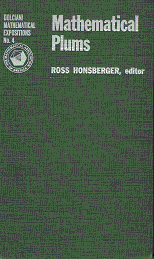## Mathematical Plums

### by Ross HonsbergerMathematical Plums is the fourth book in the Dolciani Mathematical Expositions series. The first three have been authored by Ross Honsberger; for this one, he served as an editor. The book consists of 10 chapters by various authors. The chapters are absolutely independent; some have been probably plucked by Honsberger from periodical publications, some may have been written specifically for the book. I can't say. Except for the Chapters 3, 8, and 10 by R. P. Boas that at least partly came from the MAA magazines, and Chapter 9 that grew out of the notes Honsberger took at a lecture given by A. W. Tucker, I am not aware of other sources.

Published in 1979, the book should be considered a bibliographic rarity, although in single copies it is available for just a few dollars at all major online stores. I highly recommend the book to math teachers and aspiring high school students. Honsberger has managed to put together a fine collection of articles covering a broad range of topics and written by several well known math expositors.

The chapters come with bibliographic references and many with exercises. Here is a line-up of the chapters with brief commentaries.

1. Chromatic Graphs by H. L. Dorwat and D. T. Finkbeiner

Bichromatic graphs. A. W. Goodman's formula for the minimum number of monochromatic triangles in complete bichromatic graphs. Introduction into Ramsey's thematics.

2. How to Get (at least) a Fair Share of the Cake by K. R. Rebman

Fair division. What is now called a Lone Divider Method, with a proof based on the Frobenius-König theorem. The moving knife method based on Lyapunov's theorem. Various criteria of satisfaction by the division.

3. Some Remarkable Sequences of Integers by R. P. Boas

Five proofs of the divergence of the harmonic series. Cantilevered arch. Thinning out of the harmonic series. Reciprocals of primes and of numbers with one digit left out. Distribution of the digits in integers. Integers with 1 as the leading digit. What's now called Zipf's Law.

4. Existence Out Of Chaos by S. K. Stein

Fubini principle, i.e., getting the same result in two different ways. Applies in combination with the Dirichlet principle. In number theory gives an average of the number of divisors in integers. In topology derives Euler's polyhedral formula. Additional examples from combinatorics and Latin squares.

The chapter is especially rich in exercises many of which are given helpful hints.

5. Some Surprises in Probability by Ross Honsberger

Elfron's dice. Non-transitivity paradox. George Pólya's proofreading example. Relative distribution of specific combinations in successive coin tosses. A strategy for guessing the largest number in a random sequence, with and without a known bound.

6. Anomalous Cancellation by R. P. Boas

A fascinating real math investigation prompted by the fortuitous cancellation 16/64 = 1/4.

7. A Distorted View of Geometry by G. D. Chakerian

A sample of interesting elementary mathematics that could be obtained from a few properties of the orthogonal projection; the isoperimetric inequality for triangles, for one.

8. Convergence, Divergence and the Computer by R. P. Boas

Musings on on the relevance of convergence criteria to an actual evaluation of sums partial sums by hand and on a computer.

9. Kepler's Conics by Ross Honsberger

Derivation of the equation of the cone that touches a given sphere over a circle drawn on it. An equation of the shadow conic section.

10. The Skewes Number by R. P. Boas

History and significance of the Skewes number.Mathematical Plums, by Ross Honsberger (editor). MAA, 1979, 182 pp. ISBN 0-88385-304-3.